# Bihar Board 12th Maths Important Questions Long Answer Type Part 1

BSEB Bihar Board 12th Maths Important Questions Long Answer Type Part 1 are the best resource for students which helps in revision.

## Bihar Board 12th Maths Important Questions Long Answer Type Part 1

Relations & functions

Question 1.
Show that the relation R in the set {1, 2, 3} Jut, 3} given by R = {(1, 1), (2,2), (3,3), (l, 2), (2,3)} is reflexive but neither symmetric nor transitive,
Solution:
Given Set (A) ={1,2, 3}
Given relation R = { (1,1), (2, 2), (3,3), (1, 2), (2, 3)}
∵ (1, 1), (2,2), (3, 3) ∈ R and 1,2, 3, ∈ A
∵ R is Reflexive.
∵ (1,2) ∈ R but (2, 1) ∉ R
∵ R is not symmetric. .
∵ (1, 2) ∈ R, (2, 3) ∈ R but (1.3) ∉ R
∵ R is not transitive.Question 2.
Show that the relation R in the set Z of integers given by R = {(a. b} 2 divides a – b} is an equivalence relation.
Solution:
Given set = Z (set of integers)
Given relation R = { (a, b): 2 divides a-b)
For equivalence relation.
(i) R be reflexive.
(ii) R be symmetric.
(iii) R be transitive
∵ $$\frac{a-a}{2}=\frac{0}{2}$$= 0 (Integer)
∵$$\frac{b-b}{2}=\frac{0}{2}$$ = 0 (Integer)
∴ R is reflexive.
For symmetric if a Rb
⇒ b Ra
∵ aRb ⇒ $$\frac{a-b}{2}$$ = Integer
⇒ $$\frac{-(b-a)}{2}$$ = Integer
∴ $$\frac{b-a}{2}$$ = Integer
∴ bRa
∴ R is symmetric
For transitive, If a Rb, b Rc ⇒ aRC
∵ bRb ⇒ $$\frac{a-b}{2}$$ = Integer
bRc$$\frac{b-c}{2}$$ = Integer.
Eqn. (i) + Eqn. (ii)∴ a Rc
∴ R in transitive.
Hence R is an equivalence relation.

Question 3.
Show that each of the relation R in the set A = { x ∈ Z/0 ≤ x ≤ 12} given by
(i) R – {(a, b): |a-b| is a multiple of 4.}
(ii) R – {(a, b) : a = b} is an equivalence relation. Find the set of .all elements related to 1 in each case.
Solution:
Given set A = {x ∈ R/o ≤ x ≤ 12}
(i) Relation R = {(a, b):|a- b| is a multiple of 4}.
For equivalence relation R be reflexive.
R be symmetric. .
R be transitive. .
∵ a-a = 0 = 0 x 4 = multiple of 4
b – b = 0 = 0 x 4 = multiple of 4 and so on.
∴ R is reflexive .
For symmetric, If a Rb
⇒ b Ra
Given a Rb
means |a – b| is a multiple of 4.
⇒ |(b – a)| is a multiple of 4.
|b – a| is a multiple of 4.
∴ b Ra
∴ R is symmetric.
For transitive, if a Rb, bRc ⇒ a Rc ,
Given a Rb & b Rc
=> |a – b| = multiple of 4. …………(i)
and |b-c| = multiple of 4 …(ii)
eqn. (i) + eqn. (ii) .
|a -b| + |b-c|= multiple 4.
⇒ |a – c| = multiple of 4.
⇒ a Rc
∴ R is transitive.
∴ R is equivalence relation.(ii) R = {(a,b):a = b)
For equivalence relation R be reflexive.
R be symmetric
R be transitive.
∵ Each element is equal to itself,
∵ R is reflexive.
For symmetric, if a Rb ⇒ b Ra
Given aRb
⇒ a = b
⇒ b = a
⇒ b Ra
∴ R is symmetric
For transitive, if a Rb, b Rc
⇒ aRc
Given, aRb, bRc
⇒ a = b, b = c
⇒ a = c
⇒ aRc
∴ R is transitive
∴ R is an equivalence relation.
When 1st components of each pair = 1 then
(i) R = {(1,1), (1,5),(1,9)}
∴ A={1,5, 9,}

(ii) R = {(1,1)}
∴ A = {1}

Question 4.
Prove that the greatest integer function f : R → R given by fix) – (x} is neither one-one nor on to where (x) denotes the greatest integer less than or equal to x.
Solution:
Given f: R → R.f(x) = [x].
Putx = 1.5 ∴ f(1 – 5) = [1 – 5] = 1
Putx = 1.8 – ∴ f(1 – 8) = [1 – 8] = l
∴ f is not one-one.
∵ Co-domain = R.
Rage = y =f(x) = [x] = Integral value,
∵ Co-domain ≠ Rage
∴ f is not on to.

Question 5.
Show that if f : R – – $\frac{3}{5}$ is defined by f(x) = $$\frac{3 x}{5 x} \cdot \frac{4}{7}$$ and g:R –$\frac{3}{5}$ → R $\frac{7}{5}$ is defined by f(x) $$\frac{7 x+4}{5 x-3}$$ then fog = IA and gof = IB.
Where A = R – $\frac{3}{5}$, B = R – $$\frac{7}{5}$$, IA(x) = x ∀ x ∈ A.
IB(x) = x ∀ x ∈ B.
Solution:
gof(x) = g(f(x)) = $$g\left(\frac{3 x+4}{5 x-7}\right)$$Question 6.
Let f:N → R foe a function defined as f(x) = 4x2 + 12x +15 show that f: N → S, where S is the Rage of/is invertiahte fined f-1.
Solution:
Given f(x) = 4x2 + 12x + 15
or, y = (2x)2 + 2.2x.3 + 9 + 6
or, y = (2x + 3)2 + 6
or, (2x + 3)2 = y – 6
or, 2x + 3 = $$\sqrt{y-6}$$
⇒ x = $$\frac{\sqrt{y-6}-3}{2}$$, y ≥ 6
⇒ g(y) = $$\frac{\sqrt{y-6}-3}{2}$$

∵ gof(x) = g(f(x)) = g( 4x2 + 12x + 15)
= g(12x + 3)2) + 6)= y – 6 + 6 = y
Hence gof = Infog = Is
⇒ f is invertiable with f-1 = g.

Question 7.
Let A = N x N and * be the binary operation on A defined by (a, b) * (c, d) = (a + c, b + d). Show that * is commutative and associative,. find the identity element for * on A if any.
Solution:
Given (a, b) * (c,d) = ( a + c, b + d)
For commutative, (a, b) * (c,d) = (c,d) (a,b)
L.H.S. = (a,b) * (c,d) = (a + c,b + d)
R.H.S = (c, d) * (a, b) = ( c + a, d + b) = ( a + c, b + d)
∴ L.H.S. = R.H.S.
∴ * is commutative binary operation.
For associative, (a, b) *((c, d)*(e,f)) = ((a,b) * (c, d)) * (e,/)
L.H.S. =(a,b)*((c,d)*(e,f))
= (a, b) *(c + e, d+f) = (a + c + e, b + d + f)
R.aS. = ((a, b)*(c,d) *(e, f )
= (a + c, b + d) *(e, f) = (a + c + e, b + d +f)
∴ L.H.S = R.H. S
∴ * is associative binary operation.

Algebra Matrix

Question 1.
If A = $$\left[\begin{array}{rrr} 2 & 0 & 1 \\ 2 & 1 & 3 \\ 1 & -1 & 0 \end{array}\right]$$ find A2 – 5A + 6I ?
Solution:Question 2.
If A = $$\left[\begin{array}{ll} 3 & -2 \\ 4 & -2 \end{array}\right]$$ and I = $$\left[\begin{array}{cc} 1 & 0 \\ 0 & 1 \end{array}\right]$$ k so that A2 = k.A – 2I
Solution: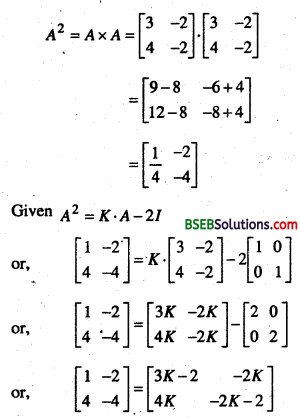∴ 3k – 2 = 1
⇒ 3k = 3
⇒ k = 1

Question 3.
A trust fund has Rs. 30,000 that must be invested in two different types of bonds, the first bond pays 5% Interest per year and the second band pays 7% interest per year. Using, matrix nmltiplicatHm determine how to divide Rs. 30,006 among the two types at bonds. It the* trust fund must obtained on arrival total interest of (a) Rs. 1800.
Solution:
Let invest in 1st bond = xRs.
∴ Invest in 2nd bond = 30,000 – x Rs.
Interest in 1st bond = 5%
Interest in 2nd bond = 7%
(a) When total annual interest of Rs. 1800 then,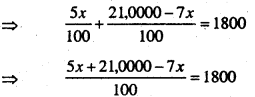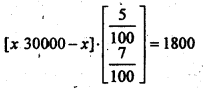⇒ -2x + 21,0000 = 18,0000
⇒ 21,0000 – 18,0000 = 2x
⇒ 2x = 3,0000
x = 15,000
∴ Invest in 1st bond = 15,000 Rs.
Invest in 2nd bond = 30,000 – 15,000 = 15,000 Rs.

Question 4.
Express the matrix A = $$\left[\begin{array}{rrr} 2 & -2 & -4 \\ -1 & 3 & 4 \\ 1 & -2 & -3 \end{array}\right]$$ as the sum of a symmetric and skew symmetric matrix.
Solution: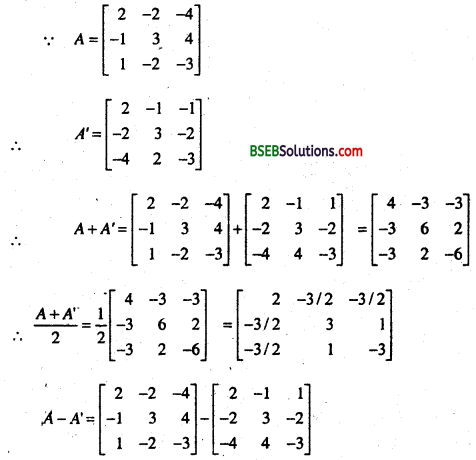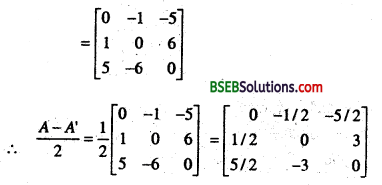∴ Sum of symmetric and skew symmetric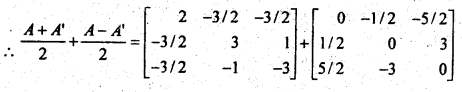Question 5.
By using elementary operatives find the inverse of the matrix A = $$\left[\begin{array}{rr} 1 & 2 \\ 2 & -1 \end{array}\right]$$
Solution:
given A = $$\left[\begin{array}{rr} 1 & 2 \\ 2 & -1 \end{array}\right]$$
∴ |A| = $$\left|\begin{array}{rr} 1 & 2 \\ 2 & -1 \end{array}\right|$$ = -1 – 4 = -5
From 1st row, co-factor of I = -1
co-factor of 2 = – 2 .
From 2nd row, co-factor of 2 = -2
co-factor of -1 = 1